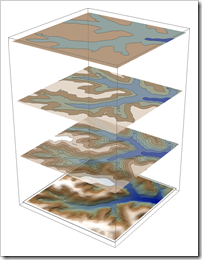## Feb 16, 2009

### Howto: Display 2D plot in 3D

In GIS field, sometimes we like to stack several 2D plots together and display them inside a 3D box. For Mathematica, we need to define a function to convert a 2D plot into a 3D graphic object. I will use a small Geotiff as an example, you can download it here if you like to try the code.

(*get the ElevationRange, then import data *)

Import["smalldem.tif", {"Geotiff", "ElevationRange"}]

data = Import["smalldem.tif", {"Geotiff", "Data"}];

Then we a create the contour plot with 100 feet contours

c1=ListContourPlot[data,MaxPlotPoints->30,Contours->Function[Range[650,850,100]],ColorFunction->”DarkTerrain”,PlotRange->{640,850}]

Here the function we need to convert 2D plot into 3D

to3d[plot_,height_,opacity_]:=Module[{newplot}, newplot = First@Graphics[plot];newplot=N@newplot /. {x_?AtomQ,y_?AtomQ}->{x,y, height} ;
newplot /. GraphicsComplex[xx__]->{Opacity[opacity], GraphicsComplex[xx]}];

This function has three parameters: 2D plot, height, and opacity

Let’s create two more contour plots with 50 and 20 feet contours respectively. Then we can stack them together by setting them in different heights.

Show[{Graphics3D[to3d[c1,30,0.75]]}, Graphics3D[to3d[c2,20,0.75]], Graphics3D[to3d[c3,10,0.75]], Lighting->"Neutral", BoxRatios->{1,1,0.8},Axes->True]

Then we like to stack the original geotiff at the very bottom. This time we need to convert the raster into 3D. I use the example you can find in Listplot3D (check the section of “Neat Examples”).

pic = Reverse[ImageData[r1]];

bg=ListPlot3D[Table[1,{x,1,800,5},{y,1,800,5}],Mesh->None, VertexColors->{pic[[1;;800;;5,1;;800;;5]]},DataRange->{{1,800},{1,800}}, Lighting->"Neutral"]

The final product:Here is the complete notebook.

AlfC said...

great post, unfortunately it doesn't work with some plots:

Show[
Graphics3D[to3d[Plot[x, {x, -1, 1}], 30, 0.75]],
Graphics3D[VectorPlot[{x, y}, {x, -1, 1}, {y, -1, 1}]],
Lighting -> "Neutral", BoxRatios -> {1, 1, 0.8}, Axes -> True]

In this case VectorPlot doesn't work, I believe it is because the arrows generated are not 3D arrows but 2D arrows that can not be interpreted by Graphics3D.

AlfC said...

answering to my own post, the following patch works with plots with arrows

to3d[plot_, height_, opacity_] :=
Module[{newplot}, newplot = First@Graphics[plot];
newplot = N@newplot /. {x_?AtomQ, y_?AtomQ} -> {x, y, height}